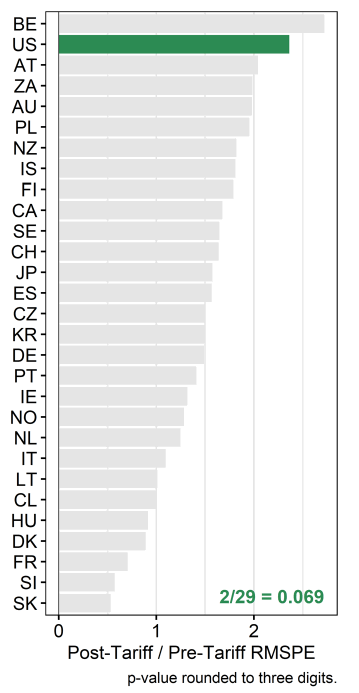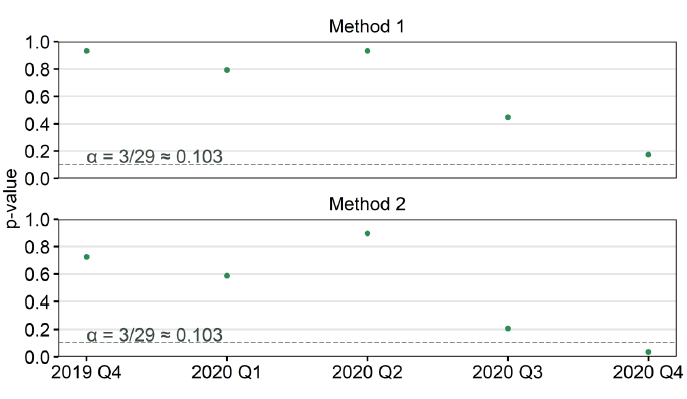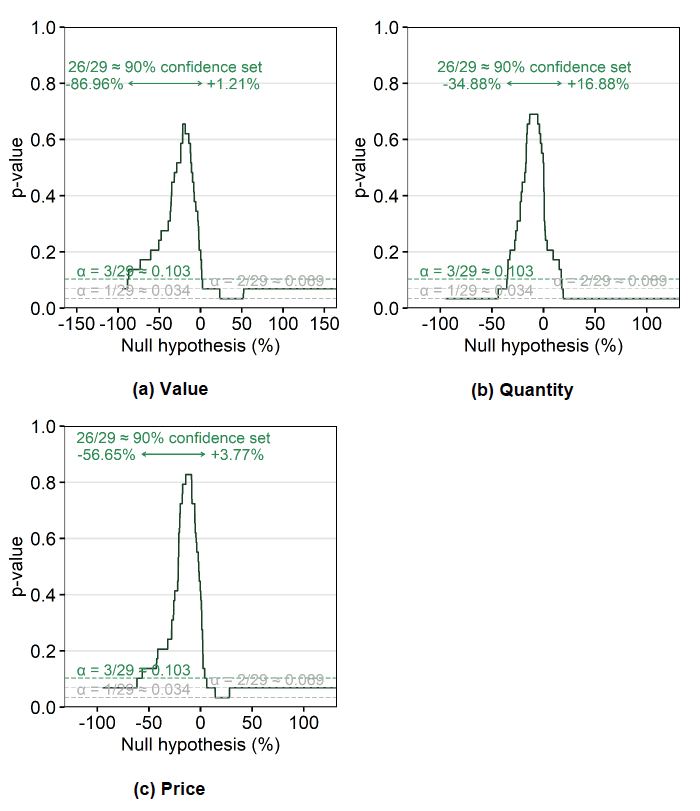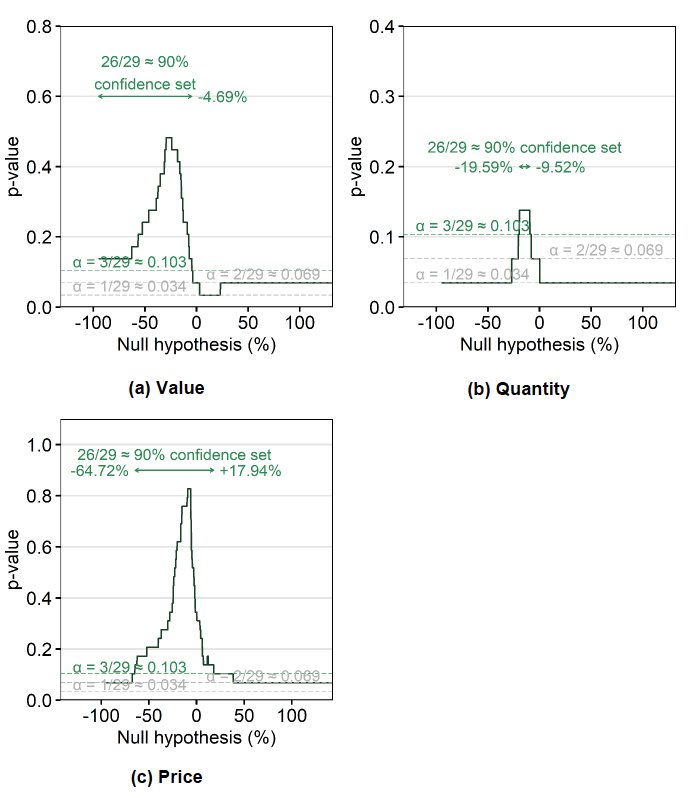# Estimating the impacts of US tariffs on UK exports of single malt Scotch whisky

This discussion paper aims to estimate the impact of US tariffs on UK exports of single malt Scotch whisky between Q4 2019 – Q4 2020 using the novel synthetic control method.

## Annex C – Inference and Confidence Sets

### Choice of Test Statistic

The ratio of post- to pre-tariff test statistic was used as opposed to the post-tariff test statistic because the pre-treatment fit for nearly all the synthetic controls' placebo tests was poor.

Using the latter option for the p-value (Section 3.2.1.1) would likely lead to restricting the donor pool to single digits. This was deemed too restrictive, and so the second option was used instead (Section 3.2.1.2). This ratio RMSPE test statistic performs well, only surpassed by the t-test statistic in the Monte Carlo studies ran by Ferman, Pinto, and Possebom (2018).

In the case of the first-lag quarterly value specification, this leads to a p-value of 0.069 (Figure C1).

This effect varies over time when looking at the absolute post-treatment gaps (i.e., root squared prediction errors) on a quarter-to-quarter (or month-to-month) basis, compared to the pre-treatment RMSPE. Figure C2 shows the p-values obtained for each quarter using either method (here dubbed 'Method 1' and 'Method 2').

This shows that the 2020 Q4 period saw particularly large deviations from the synthetic control (due to the tariff or some other US-specific deviation compared to the synthetic control) using the ratio test statistic ('method 2').Figure C1. The ratio of post- to pretariff fit for the first-lagged quarterly value specificationFigure C2. P-values obtained from the post-tariff RMSPE test statistic (upper) and ratio RMSPE test statistic (lower) by post-tariff quarter

### Impact Estimates

The estimated tariff impact is given by the post-treatment gap (of averages) less the pre-treatment gap (of averages). Since the dependent variable is in logged per-capita terms, these averages are calculated as follows:

 Pre-tariff: Post-tariff:

where is the observed export value, is the counterfactual export value (synthetic control), and and refer to the pre-treatment and post-treatment periods.

Were we to calculate proportional differences using averages of the original per-capita values (or total values), we'd arrive at slightly different proportions. Since the above logged proportional differences are also used for hypothesis testing (by adding or subtracting to the left-hand-side of the post-tariff equation above), the other proportional differences are not explored.

### Confidence Sets

Two-sided confidence sets were constructed using the test statistic described in Section 3.2.2. Results for (a) value, (b) quantity, and (c) price are shown below (using a first-lag specification) for both the monthly and quarterly specifications.Figure C3. 26/29 confidence sets for the first-lagged monthly specifications with value, quantity, and price as dependent variablesFigure C4. 26/29 confidence sets for the first-lagged quarterly specifications with value, quantity, and price as dependent variables

### Contact

Email: agric.stats@gov.scot